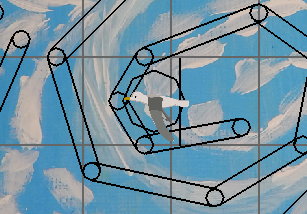Timothy Barnes
Recently I made a system for determining how the wind paths in the game will be placed within a scene.

Wind paths are the air currents or gusts that the player will need to navigate through to stay with other characters.

Due to the nature of how I plan to use these paths in the game, it is necessary that the layout for each wind path is chosen dynamically depending on what is happening in the game.

One approach I have found useful for creating the layout is to generate a chain of circles in which each circle is touching another circle's perimeter. A number of points are then chosen consecutively along the perimeter of the circle chain to describe the curved path. Straight line segments can also be part of the chain to provide variation in the path.

One of the benefits of this approach is that the circles and line segments that define the chain can be used as geometric primitives for overlap testing. This is necessary for determining if one path is overlapping another path. Testing using the chain is much faster than comparing the individual line segments of two paths for overlap.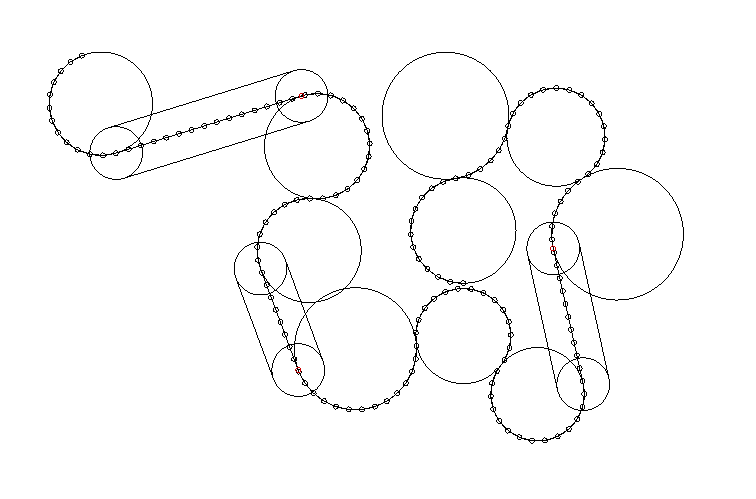In this picture the wind path is shown as a dotted line following the perimeter of the circles in the layout. Straight line segments are represented as a capsule extending from one circle to the next.

The next task is to design the character behavior involving the wind paths. In the game a goal is to stay with other birds and avoid losing them in the wind. Both the behavior of the wind paths and the behavior of the other characters is hoped to make this goal challenging.Timothy Barnes
Introduction to Series
This is the fourth part in a series about programming with splines. Part 1: Introduction, Part 2: Closest Point, and Part 3: Arc Length can be found at those links.

Introduction to Post
The gist of what I will be explaining today is the problem of inconsistent speed when moving down a spline. This happens when the spline’s control points are at different arc lengths from each other. This can occur when the control points have been specified by a person using a game’s level editor, or the control points are from a path imported from a digital content creation tool. I will show an approach to this problem I learned from a paper titled “Arc-Length Parameterized Spline Curves for Real-Time Simulation”, that involves generating a new spline path of control points at equal arc length along the spline. I will also briefly discuss some problems with this approach.

Introduction to the Problem
If we linearly increase the spline parameter from the spline interpolation equation we discussed in part 1 each frame, there is no guarantee that the interpolated point will be moving at a constant speed. It could speed up or slow down significantly depending on how close the control points are to each other.

For instance, if we used the elapsed time since the application started running as the spline parameter, then within one second the interpolated point would transition from control point 0 to control point 1. At the end of the second second, it would be on control point 2, and so on. In this case it takes one second to move to each new control point, regardless of the distance between the control points. If the next control point is very far away, then the interpolated point will appear to move quickly. If a control point is near to a previous one, the interpolated point will appear to move slowly.

What we would like to do is write our program so that an interpolated object does not hit these snags and speedy places, but instead will move at a constant speed.

There are times when you want to vary the speed, but you first need to be able to interpolate smoothly down the spline at a constant speed in order to have complete control of the speed. For example, if you were writing a single player racing game, you might program the other drivers to move down the track on a spline path. In that example it might be a good idea to vary the speed of the other drivers so that they slow down when going around a tight curve. If you did not start with a spline that moved at a constant speed, then you would be at the mercy of however close or far away the next control point would be.

Numerical Methods
The numerical methods approach to the problem of moving at a constant speed down the spline is to write a function that takes in a parameter for the distance along the arc length that you want the interpolated point to be, and that has an output of the correct spline parameter for that given arc length.

Once the spline parameter is found it can be passed to the spline interpolation function we previously discussed to find a point at a given arc length down the spline. Unless a lookup table is generated, this approach relies on real-time numerical methods, which bring along all the host of problems we have previously discussed (variable execution time, no promise of convergence).

Generating an Equally Segmented Spline
Despite that this problem is often solved using numerical methods, a different approach exists that is more stable due to not relying on real-time numerical methods. I learned this other approach in a paper by Wang, H., Kearney, J., and Atkinson, K.  I call it the “equally segmented spline” approach because it involves generating a new spline in which each segment has an equal arc length.

This approach works by generating a new spline with different control points. These control points are chosen so that they lie on the path of the old spline, but are at an equal arc length from each other. Using this approach, you would pass the desired arc length parameter into the interpolation function for the new spline. If you generated the spline so that the control points are each at one world unit from each other, then you now have the point at your given arc length along the spline. Otherwise the parameter to the interpolation function would need to be scaled based on the chosen set distance between the control points. This relied on no real-time numerical methods, and the computation cost is identical to interpolating on any other spline.

Problems with the Equally Segmented Spline Approach
This approach relies on preprocessing a spline curve before an object is able to move at a constant speed down the path. This needs to be taken into account as to the technical complexity it might bring to a project. If the control points in a spline are in constant motion, then the new spline would need to be regenerated each frame before being used for interpolation. This could be prohibitively expensive.

The other problem involves choosing the number of control points for the new spline. If too few control points are chosen for the new spline, then the new spline will not approximate the old spline well. The worse case is when the original spline pinches tightly or forms a loop like a roller coaster. If the loop is one meter along the arc length, and the distance you have chosen between all the new control points is also one meter, you could end up having two control points directly next to each other, one at the start and one at the end of the loop. Since the new spline has no middle control point between the two, there will be no loop there. The control points may end up not being at and equal arc length from each other after all!

Conclusion
The best method for moving smoothly at a constant speed down a spline depends on the problem you are trying to solve. If you have tried using numerical methods for this purpose and found it lacking, give the equally segmented spline approach a try. If you know of other approaches to this problem, please let me know and post in the comments below.

 Wang, H., Kearney, J., and Atkins...e for real-time simulation (2002)Timothy Barnes
Introduction
In this post I will give an overview of how I arrived at the current movement system for Seabird and some of the challenges that I faced. I will show the problems with my initial idea of using a vector grid, and how my use of cubic splines largely replaced that. Then I will discuss the reasons for going back to a vector-based approach, and the flexibility that it has given me for scene layout.

This video shows what the character and wind movement presently looks like:

(Note: This scene is made of placeholder art. The player is marked by a circle.)

Requirements for a Movement System
Most of the gameplay in Seabird involves trying to avoid becoming separated from other birds in scenes made up of wind currents. If the player loses sight of a bird, they may need to find another bird that is willing to help them.

The only gameplay requirement for the wind currents is that they present some challenge to the player for staying with other birds. Two approaches I used for this are:
- Once a bird enters a wind current it is difficult to leave the current.
- Wind currents can move in the scene.

Vector Field
The first approach to tackling this problem I tried was to represent the entire scene as a vector field, or vector grid. For every point in the scene, there was a force vector for the wind force at that point. This worked well, except that I did not have a good way to set up the forces at each point without doing it manually. I experimented with different functions to set up the scene, but I could not find an approach to this that was satisfying.

An approach I could have tried would have been to use to the level editor to “paint” the vector grid. One problem I would have needed to work around if I chose to do this would be how I would make currents that are animated.

Direct Movement on Spline
Then I started looking into using parametric paths for the wind currents. Instead of a vector field for the entire scene, I set up the scene using multiple spline paths. I found that splines were a good fit for the problem I was trying to solve. Splines are much nicer to work with, and provided a more straight forward way to both manually and procedurally set up the wind currents in the scene. They are also easy to animate and move around in the scene.

Instead of using vectors, I tried to write the movement code using only spline mathematics. With a spline, it is easy to move a point along the path at a constant speed. This speed can be adjusted by whether the player’s input vector (direction of joy-stick, or arrow keys) is facing more upstream or downstream on the path, as well as the curvature of that segment on the path. The player is also able to get off the path by facing the input vector orthogonality to the spline slope.

Diverging Paths and Problems with Direct Movement
The major problem I ran into with this technique was that it became difficult to handle the case in which multiple wind currents might be affecting the player.

For instance, how should I handle the case in which a wind current forks into two currents, or in which a smaller current branches off of the first? In these cases, two or more wind paths need to be influencing the player. Moving between them smoothly was difficult. I worked out a solution, but I was not satisfied with it so I moved on to another approach.

Vector Spline Movement
I decided to go back to the vector field approach but instead determine the vector forces by the spline paths in the scene. The idea is this: when updating each of the characters, find a number of points nearby and determine a wind vector for each point based on the spline paths in the scene. Get an average of these points, and let that be the wind force applied to the character each frame.

To make it difficult for the birds to leave the wind current, I set wind vectors near the edge of the path to point strongly inward. Branching of wind paths was now a trivial problem. For a given point I add up the vectors of the wind for each wind path.

Conclusion
Each solution seems obvious to me now that I have seen it working. At the time it was difficult to know what would work and what would not. If some unseen challenges come up, I may need to again rethink this system, but for now it seems like a mostly viable solution to ship in the game.Timothy Barnes
Introduction to Series
This is the 3rd post on splines in a series I am writing primarily for game programmers and others interested in the basics of using splines in their software.
In part 1 of this series I wrote an introduction to cubic splines. In part 2 I showed one way for how to find the closest point on a spline path to another point in the world.

Introduction to Post
Today I will discuss the problem of finding the length of a spline. I will go into why it is useful and a little bit of the mathematics behind it. Afterwards I have some example code for an approach to finding the arc length. I also briefly discuss other approaches that may improve the accuracy of computing the length.

What is the Arc Length?
When people discuss length in the context of a spline, they usually mean the arc length. This is how long the spline would be if it were stretched out without any curves. You can also think of this as the distance you would need to travel if you walked along the spline from the beginning to end.

Why is it Useful?
When programming a game that uses splines, it is useful for many reasons to know the arc length. In a city-building game the amount of road the player places down is proportional to how much it costs the player. If the road were represented as spline paths, then the arc length would be used to determine the total cost. In a racing game, the arc length could represent the length of the track if the programmer had chosen to represent the track as a spline path.

How to Specify it Mathematically
The ordinary distance between two points p and q in 2D is: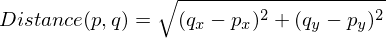We can approximate the arc length of the spline by finding points along the spline, and adding up all of the lengths between each consecutive point. If we subdivide the spline path into smaller and smaller segments, then the approximation more closely matches the true arc length. To say it another way, the limit of the sum of lengths between consecutive points, as the number of points approaches infinity, is the arc length.

Mathematically this is represented as an integral over the spline. It can be written like this: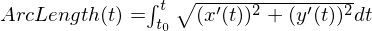The functions x and y are the dimensional functions for the spline in 2D. The variable t is the spline parameter. For a cubic spline, these functions are written below: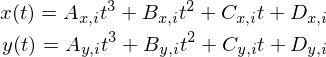A_xi is the first spline coefficient in the x dimension on spline segment i.

Code Example
The simplest way to find the arc length for a spline in code is to choose a number of points along the spline, and add up the lengths between each consecutive point. One way to write this code:
  1 2 3 4 5 6 7 8 9 10 11 12 13 14 15 16 17 18 19 20 21 22 23 24 25 26 27 28 29 30 31 32 33 34 35 36 37 38 39 40 vec2 SplineSegment(vec2 a, vec2 b, vec2 c, vec2 d, float x) { vec2 result; // ax^3 + bx^2 + cx + d result.x = x*(x*(a.x*x + b.x) + c.x) + d.x; result.y = x*(x*(a.y*x + b.y) + c.y) + d.y; return result; } float SplineTotalArcLength(const cubic_spline *spline) { float totalLength = 0.0f; for(int segment = 0; segment < spline->controlPointCount; segment++) { const float granularity = 16.0f; // Integration samples per spline segment. vec2 last = spline->coefficients[segment]; float segmentLength = 0.0f; for(float i = 1.0f; i <= granularity; i += 1.0f) { float x = i / granularity; vec2 next = SplineSegment(spline->coefficients[segment], spline->coefficients[segment], spline->coefficients[segment], spline->coefficients[segment], x); float subsegmentLength = Length(next - last); last = next; segmentLength += subsegmentLength; } totalLength += segmentLength; } return totalLength; } 

Other Techniques
This solves the problem of finding the arc length, but it is not necessary the most accurate algorithm to do the job. While it will still closely approximate the arc length of the spline if the number of points is sufficiently large, there are other methods that give more accurate results with the same number of control points

Since we have framed this problem as an integral over the spline path, we can use other conventional numerical analysis techniques to solve the integral. These other approaches are beyond the scope of this article. For those interested, the techniques to look up are Simpson’s Rule or Gaussian quadrature.

Conclusion
I showed how finding the arc length is applicable to many challenges game programmers may face when working with splines, as well as a straightforward approach to finding the arc length. In the next article I will discuss a technique for getting smooth constant velocity movement along a spline path without relying on numerical analysis techniques that need to run in real-time.Timothy Barnes
This is the second part in a series on using splines for computer games and other real time applications. In this post we will briefly go over how we define the closest point and why we want to find it. Then we will more formally state the problem we are trying to solve. There is a short overview of numerical analysis and an approach called Newton’s method, and I will explain some of the problems with the method as well as a few ways to get around those problems.

(Note: if you are familiar with numerical analysis, skip to the "2 Strategies for Initial Guess" section.)

How to Think About the Closest Point on a Spline
One way to think about the problem of finding the closest point on the spline is to imagine that the spline represents a road. We are trying to find the place along the road where the distance from the road to another object on the landscape (let’s say a windmill) is as small as possible. The road may twist back and forth many times. It could approach close to the windmill at numerous points along its length. How do we find which point is the closest? One solution to this problem is to take tiny steps along the road and take a measurement from the road to the object at each step. After walking across the entire road, you can then determine which step was the closest.

Reasons for Finding the Closest Point
Finding the closest point on a spline to another point in the world can be useful for many reasons. Once this point is found, it is easy to determine whether a point or sphere is colliding with any part of the spline path. In Seabird I find the closest point on the spline to one of the birds to determine whether that bird has entered a wind path.

Equation we are Trying to Minimize
Let’s be a little bit more formal with defining our problem. As discussed in the last post, we define the spline using a cubic polynomial with coefficients A B C D. We will use the squared distance between the spline and a point P in the world.We want to find a parameter x, such that that L as small as possible. This parameter needs to be the smallest that we can find on any segment of the spline.

Minima and Maxima
The type of problem we have is called an optimization problem. If you think of a mathematical function like a landscape, it may have many dips and valleys, as well as hills and mountains. The lowest point in the lowest valley is called a global minimum. A hole in the road might not be the lowest point in a city, but water will still fill the hole when it rains. This is called a local minima (as in the plural form of minimum). This is an important concept, because as we will discuss, it is easy for algorithms to accidentally mistake a local minima for a global minimum.

Short Intro to Newton’s Method
The most common approach to solving minimization problems is a technique called the Newton-Ralphson method, or Newton’s method for short. The idea behind this method is to start with some initial guess for the parameter of the equation, and iteratively refine the guess until we arrive at some value that is hopefully closer to the value we want. The value we are trying to find in Newton’s method is the root of the equation. This is the point where the equation has a value of 0. For instance, in the above equation L would be zero if D were equal to P and the parameter x were 0. An equation may have many roots, or none at all! Because of this, careful use of Newton’s method is necessary to achieve desired results.

Using the Derivative of our Equation
We would like to use Newton’s method to find the minimum of the squared distance equation listed above. However, the squared distance equation we have will not work because it will have no root if the point does not happen to lie directly on the spline. If we are not on the spline, the minimum distance is always greater than zero. And yet, all is not lost! If we take the derivative of the squared distance equation, we can use the fact that there is a root for the derivative at every point where the squared distance equation has a minima and maxima.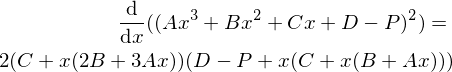Why Newton’s Method is Lousy here
Using the derivative here with Newton’s method becomes troublesome. The derivative indeed has roots, but it has too many roots! This is the problem discussed earlier. Where there are many local minima Newton’s method is likely to get stuck, preventing it from ever finding the global minimum for the function. Not only that, but it will even get stuck at the maxima, because those points on the derivative also happen to be a root.

2 Strategies for Initial Guess
There are 2 main strategies I use for avoiding the problem with Newton’s method getting stuck in local minima and maxima for splines. In the first approach we simply do a linear search over the whole spline, and find which sampled point has the smallest value, and then we run Newton’s method at that point for finer precision.

The second approach is to use the spline parameter we have found from the previous frame for the initial guess on the current frame. For instance, once the bird is moving along the wind path, it is highly likely that the previous parameter is a very good starting guess for the next frame. However you happen to get the initial guess, it is important that the guess is reasonably close to the global minimum.

Using Newton’s Method
Just before we dive into the code, I want to get a little bit more in depth with newton’s method. There is plenty of literature already available for an introduction to how it works. The method is defined like this: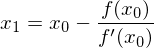The function f is the function for which we are trying to find a root. We also need f prime, the derivative of the equation to use as the denominator.
Since we are trying to find the root of the derivative of the squared distance equation, we need to also find the second derivative of the square distance equation so we can use it in Newton’s method as the denominator in the equation.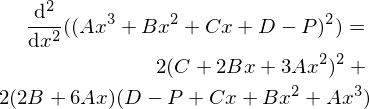Here is some example code showing the technique for finding the global minimum by first doing an initial linear search over the spline. First we need the spline segment squared:

 1 2 3 4 5 6 float SplineSegmentSquared(vector A, vector B, vector C, vector D, float x) { // (Ax^3 + Bx^2 + Cx + D - P)^2 float segment = A*Power3(x) + B*Power2(x) + C*x + D; return segment*segment; } 

We can then do a consecutive search over the spline to find a rough approximation to the global minimum:

  1 2 3 4 5 6 7 8 9 10 11 12 13 14 15 16 17 18 19 20 21 22 23 24 25 float approximateClosestParam = 0.0f; float minDistance = FLT_MAX; const float searchGranuarlity = 0.2f; for(float i = 0; i < (float)controlPointCount; i += searchGranuarlity) { int segmentIndex = (int)i; // integer part float param = i - (float)segmentIndex; // decimal part float distance = SplineSegmentSquared(coefficients[segmentIndex], coefficients[segmentIndex], coefficients[segmentIndex], coefficients[segmentIndex]-point, param); if(distance < minDistance) { minDistance = distance; approximateClosestParam = i; } } 

approximateClosestParam will be the rough estimate for the parameter along the spline where it is closest to the point. We can then approximate the parameter using Newton’s method. First we need to implement two more equations, the derivative and second derivative of the spline segment squared:

  1 2 3 4 5 6 7 8 9 10 11 float SplineSegmentSquaredDx(vector A, vector B, vector C, vector D, float x) { // Derivative of ((Ax^3 + Bx^2 + Cx + D)^2) return 2 * (C + x*(2*B + 3*A*x)) * (D + x*(C + x*(B + A*x))); } float SplineSegmentSquaredDx2(vector A, vector B, vector C, vector D, float x) { // Second derivative of ((Ax^3 + Bx^2 + Cx + D)^2) return 2 * Power2(C + 2*B*x + 3*A*Power2(x)) + 2*(2*B + 6*A*x) * (D + x*(C + x*(B + A*x))); } 

(Note: that I am using a power of 2 on a vector here. This is assumed to be component wise (V.x*V.x, V.y*V.y, etc...)

Then we can run Newton’s method using approximateClosestParam as the initial guess:

  1 2 3 4 5 6 7 8 9 10 11 12 13 14 15 16 17 18 19 20 21 22 23 24 25 26 27 28 29 30 31 const float acceptableError = 1.0e-4f; const int maxIterations = 3; float guess = approximateClosestParam; for(int iteration = 0; iteration < maxIterations; iteration++) { int segmentIndex = (int)guess; // integer part float param = guess - (float)segmentIndex; // decimal part float numerator = SplineSegmentSquaredDx(coefficients[segmentIndex], coefficients[segmentIndex], coefficients[segmentIndex], coefficients[segmentIndex]-point, param); float denominator = SplineSegmentSquaredDx2(coefficients[segmentIndex], coefficients[segmentIndex], coefficients[segmentIndex], coefficients[segmentIndex]-point, param); if(fabs(numerator) < 1.0e-4) break; // found root if(fabs(denominator) < 1.0e-6f) break; float newGuess = guess - (numerator / denominator); guess = newGuess; } 

Conclusion
Newton’s method is a simple iterative approach; however, it has weaknesses which are particularly evident when working with splines. We can get around these weaknesses with a sufficiently good initial guess; however, it is difficult to determine how good the guess needs to be in a specific case. If you have found a better approach to this problem that works for you, please let me know.

I intended this to be a 3 part series, but it looks like the series will end up being 4 posts. In part 3 I plan to show how to get the total length of the spline. In part 4 I will go over an approach to reparameterizing the spline. After we have done the reparameterization, we can move smoothly along the spline at a continuous speed. This is very useful if we want to control the speed of an animation or motion with spline independently of the shape of the spline. The approach I will discuss has zero real time cost, which makes it appropriate for this blog series.

Resources
Wolfram Alpha has an online derivative solver.
https://www.wolframalpha.com/inpu...of+Ax%5E3+%2B+Bx%5E2+%2B+Cx+%2B+D

A paper to look at for further work on the problems we discussed is Wang, H., Kearney, J., and Atkinson, K., Robust and Efficient Computation of the Closest Point on a Spline Curve.
The approach discussed in this paper combines Newton’s method with quadratic minimization to achieve a better result.
http://homepage.divms.uiowa.edu/~.../CurvesAndSufacesClosestPoint.pdf